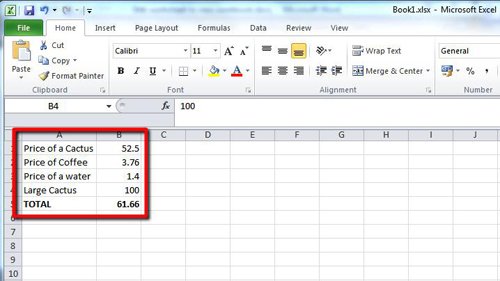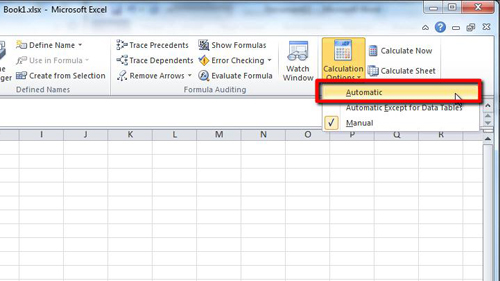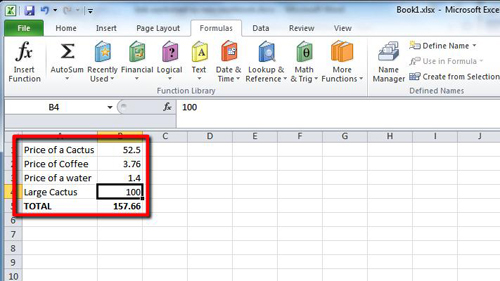## How to Make Excel 2010 formulas calculate automatically

The default setting is for formulas to automatically calculate in Excel. This tutorial will show you how to make excel formulas calculate automatically if this setting has become deactivated.

Step # 1 – Opening a Simple Spreadsheet

Start MS Excel and load a sheet with some information. Here you can see some price information about some simple goods and a total row utilizing the SUM formula. Normally when we change one of the values, the “TOTAL” will change as the SUM formula will update it automatically. Here we can see that this is not happening, even when we change a value to “100”.Step # 2 – Switching on Automatic Formulas

To enable the automatic calculation of formulas you need to click on the “Formulas” tab in the main menu, from here you need to go to the “Calculation” area and click on the “Calculation Options” icon, in the drop down list click on the “Automatic” option.Step # 3 – Seeing the Changes Applied

As formulas will now be calculating automatically you will see the “TOTAL” column automatically adjust to a larger number as the SUM formula takes into account the new figures. From this point on all formulas will calculate on their own and that is how to make excel formulas calculate automatically.